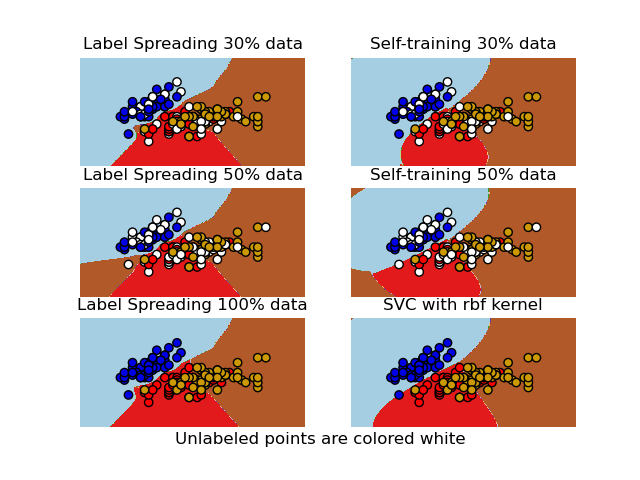# Decision boundary of semi-supervised classifiers versus SVM on the Iris dataset¶

A comparison for the decision boundaries generated on the iris dataset by Label Spreading, Self-training and SVM.

This example demonstrates that Label Spreading and Self-training can learn good boundaries even when small amounts of labeled data are available.

Note that Self-training with 100% of the data is omitted as it is functionally identical to training the SVC on 100% of the data.print(__doc__)

# Authors: Clay Woolam   <clay@woolam.org>
#          Oliver Rausch <rauscho@ethz.ch>

import numpy as np
import matplotlib.pyplot as plt
from sklearn import datasets
from sklearn.svm import SVC
from sklearn.semi_supervised import SelfTrainingClassifier

X = iris.data[:, :2]
y = iris.target

# step size in the mesh
h = .02

rng = np.random.RandomState(0)
y_rand = rng.rand(y.shape)
y_30 = np.copy(y)
y_30[y_rand < 0.3] = -1  # set random samples to be unlabeled
y_50 = np.copy(y)
y_50[y_rand < 0.5] = -1
# we create an instance of SVM and fit out data. We do not scale our
# data since we want to plot the support vectors

# the base classifier for self-training is identical to the SVC
base_classifier = SVC(kernel='rbf', gamma=.5, probability=True)
st30 = (SelfTrainingClassifier(base_classifier).fit(X, y_30),
y_30, 'Self-training 30% data')
st50 = (SelfTrainingClassifier(base_classifier).fit(X, y_50),
y_50, 'Self-training 50% data')

rbf_svc = (SVC(kernel='rbf', gamma=.5).fit(X, y), y, 'SVC with rbf kernel')

# create a mesh to plot in
x_min, x_max = X[:, 0].min() - 1, X[:, 0].max() + 1
y_min, y_max = X[:, 1].min() - 1, X[:, 1].max() + 1
xx, yy = np.meshgrid(np.arange(x_min, x_max, h),
np.arange(y_min, y_max, h))

color_map = {-1: (1, 1, 1), 0: (0, 0, .9), 1: (1, 0, 0), 2: (.8, .6, 0)}

classifiers = (ls30, st30, ls50, st50, ls100, rbf_svc)
for i, (clf, y_train, title) in enumerate(classifiers):
# Plot the decision boundary. For that, we will assign a color to each
# point in the mesh [x_min, x_max]x[y_min, y_max].
plt.subplot(3, 2, i + 1)
Z = clf.predict(np.c_[xx.ravel(), yy.ravel()])

# Put the result into a color plot
Z = Z.reshape(xx.shape)
plt.contourf(xx, yy, Z, cmap=plt.cm.Paired)
plt.axis('off')

# Plot also the training points
colors = [color_map[y] for y in y_train]
plt.scatter(X[:, 0], X[:, 1], c=colors, edgecolors='black')

plt.title(title)

plt.suptitle("Unlabeled points are colored white", y=0.1)
plt.show()


Total running time of the script: ( 0 minutes 2.020 seconds)

Gallery generated by Sphinx-Gallery